# Algorithms, equivalence of

(diff) ← Older revision | Latest revision (diff) | Newer revision → (diff)

A binary relation connecting algorithms of a fixed type and expressing the fact that any two algorithms thus connected yield the same results if fed with the same given type of inputs (and may also yield some additional information as regards the computations thus performed — the so-called history of the computation). A few typical examples of this connection are given below.

a) Consider all possible recursive schemes — i.e. systems of equations which define-place partial recursive functions. Two schemes which define functionsandare called functionally equivalent if for any natural numbers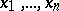the conditional equality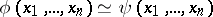is true (it is considered to be true if both its sides are meaningful at the same time and, if meaningful, assume the same value). It was shown by S.C. Kleene (see, for example, ) that for any everywhere-defined computable operator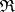over recursive schemes it is possible to find a schemesuch thatand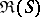are functionally equivalent (the so-called fixed-point theorem). The consequence of this is, in particular, the Uspenskii–Rice theorem on the unsolvability of any property of recursive schemes which is invariant with respect to functional equivalence if there exist schemes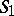and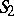such thathas this property, while schemedoes not have it. The unsolvability of the relation of functional equivalence itself follows from this theorem.

b) Consider normal algorithms (cf. Normal algorithm) over a fixed alphabet. Two such algorithmsandare called equivalent with respect toif for any word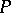inthe following condition is satisfied: If one of these algorithms convertsto some word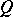which is also contained in the alphabet, then the second algorithm also convertsto. These algorithms are called completely equivalent with respect toif for anyinthe conditional equalityis true. Both these relations are unsolvable.

c) Consider logical schemes of algorithms (Yanov schemes, ). The realization of such a scheme is a sequence of operators involved in its execution, from beginning to end. Two schemes are called equivalent if the sets of their realizations coincide. The equivalence relation of Yanov schemes proved to be solvable, and a complete system of equivalent transformations has been constructed for it , .

A detailed study of the relations of algorithmic equivalence is of major importance in the context of a number of tasks of current interest (especially in the theory of programming schemes), which can only be solved by advanced techniques of equivalent transformations of algorithms. Such problems include the translation of algorithms from one language to another and their optimization (transformations aimed at improving their "working characteristics" ). Special attention is given in this connection  to finding solvable relations of algorithmic equivalence which would permit complete systems of equivalent transformations that are convenient with regard to their applications. The concept outlined in  to a large extent determined the general approach to the study of algorithmic equivalence.

How to Cite This Entry:
Algorithms, equivalence of. Encyclopedia of Mathematics. URL: http://encyclopediaofmath.org/index.php?title=Algorithms,_equivalence_of&oldid=12948
This article was adapted from an original article by N.M. Nagornyi (originator), which appeared in Encyclopedia of Mathematics - ISBN 1402006098. See original article